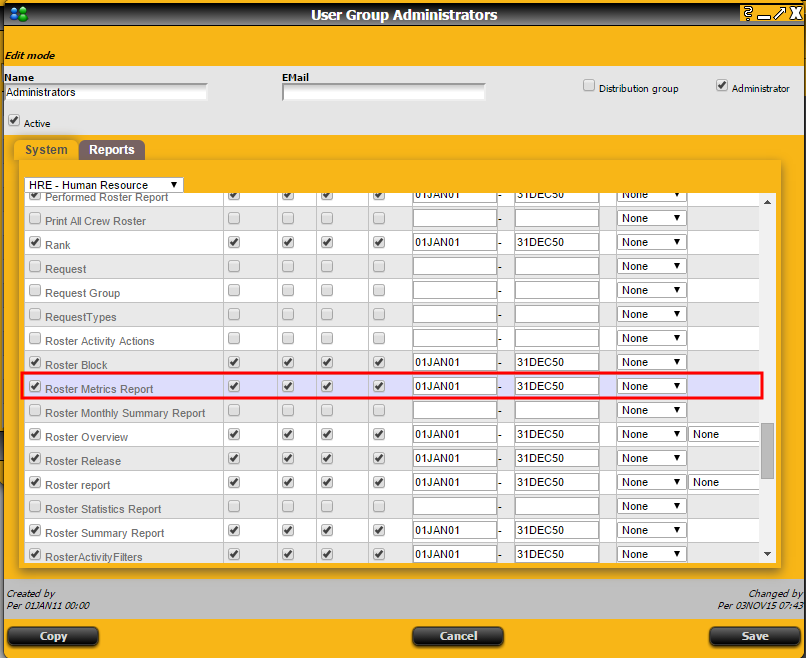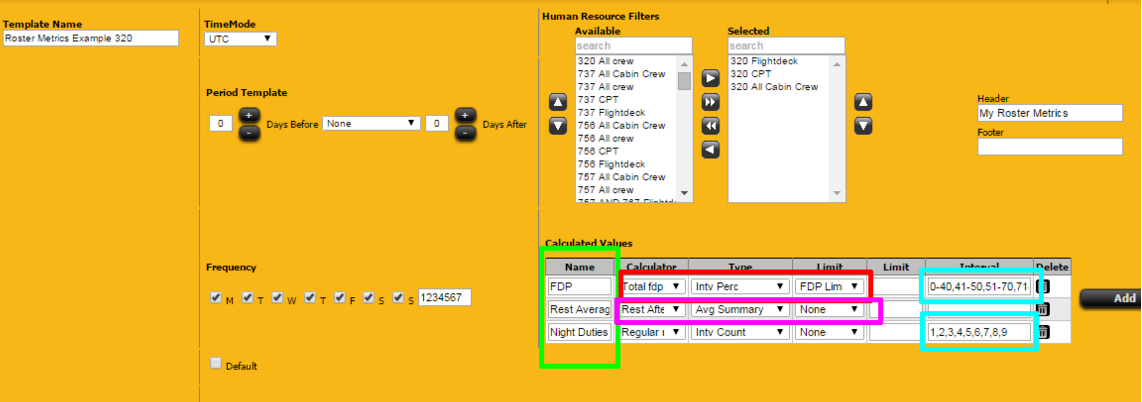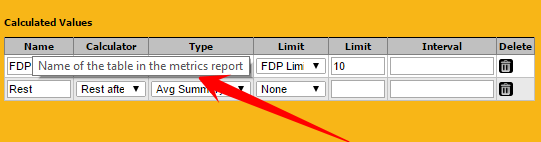# Roster Metrics ReportTo activate the Roster Metrics report in your User groups:The Roster Metrics is a report that generates a report consisting of tables. These tables have values depending on the user settings in the report template. The report checks legality on the chosen crew groups (divided by filters), and uses the values from legality to make various calculations (as set up in the template). The table in the grid needs to be populated for the report to be generated correctly.

The following fields exist:
Name: (Light green rectangles in the picture below)  - This will be the name of the Table generated in the report.
Calculator: These are the public calculators set up in Legality. The calculator will be the basis for the data presented in the report.
Type: This is the type of calculation that should be made on the calculators.  Here are the current calculators:
Interval Perc: Example: If the user wants to see a percentage of the FDP vs FDP Limit, it can then be presented in intervals - (see intervals below).
Interval Count: Put the values in different intervals. For example, this could be the number of consecutive nights, or Deep Early Starts etc.
Average Summary: The values is shown as an average value for the calculator. Example: The 'Rest At End' Calculator can show the average rest for any given crew group (see the purple rectangle below)
Summary: Will present a summary of all values taken from the Legality Calculator.
"Limit" - (Calculator Limit): Setting this calculator:  it will validate the above set calculator with this one, as a percentage. This is useful, for example, when you want to compare crew members FDP vs FDP Limit. (Red rectangle).
Interval :  (Blue rectangles): This is set if the result will be presented in intervals. e.g. Number of Consecutive nights, can be shown in intervals 1,2,3,4 etc, to see how many of these occur in the given period for the selected group. But it can also be set like this: 1-10,11-30,31-50,51-100 if the "Interval 'Perc" is used as 'Type'. Then it will show these values in the percentage groups, set in the intervals.There are various mouse-over tool-tip information in each fields, as shown below:There is also an option, called "Interval Summary Count", which will accumulate all values for each crew. This means that the report will show the total values for each crew, instead of reporting
for each roster activity.

Example: Night Duties, if you have set up an interval 1,2,3,4,5,6,7,8,9

If one crew has 3 night duties, this value will add up the value in the column called 3 in the report, (if this new interval option is chosen).

Did you find it helpful? Yes No

Send feedback